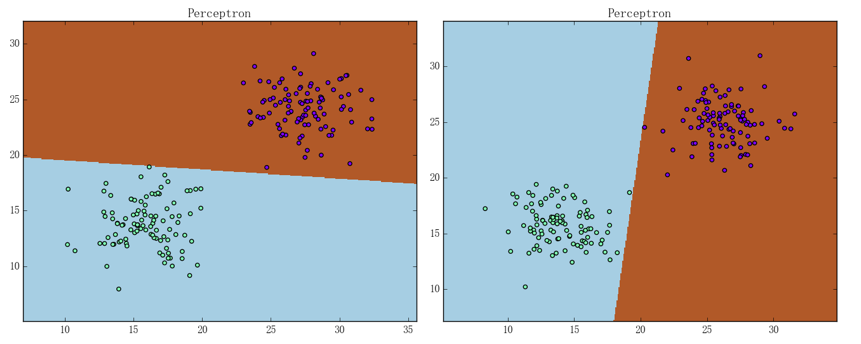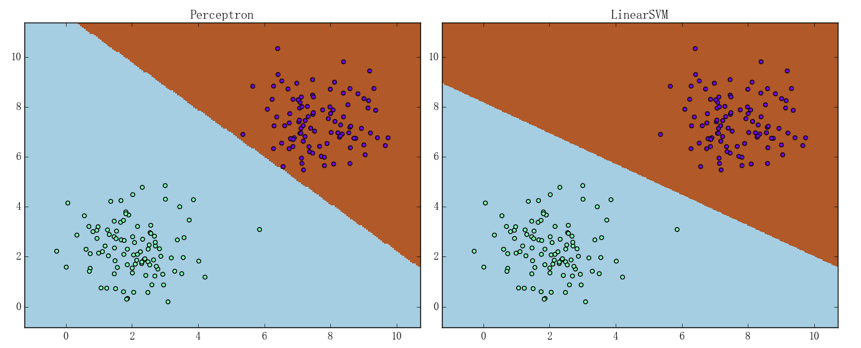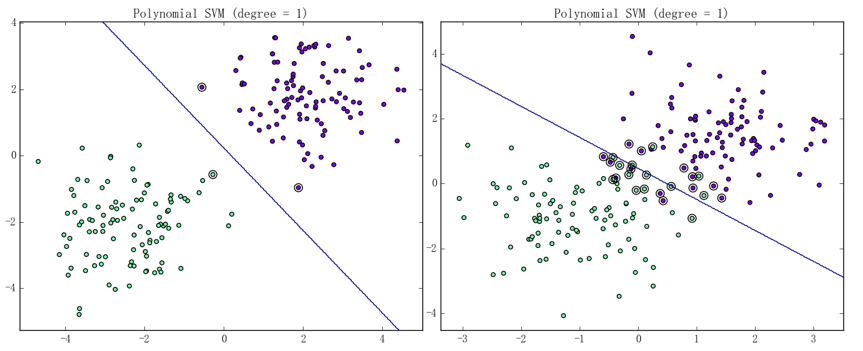# 间隔最大化与线性 SVM

$D$的最大硬间隔分离超平面。之所以称它为“硬间隔”的理由会在后文叙述，这里暂时按下不表。需要指出的是，考虑到优化问题中的不等式约束、易知在超平面

1. 输入：训练数据集$D = \{\left( x_{1},y_{1} \right),\ldots,\left( x_{N},y_{N} \right)\}$、迭代次数 M、惩罚因子$C$、学习速率$\eta$，其中：
2. 过程
1. 初始化参数：
2. $j = 1,\ldots,M$
1. 算出误差向量$e = \left( e_{1},\ldots,e_{N} \right)^{T}$、其中：
2. 取出误差最大的一项：
3. $e_{i} \leq 0$则直接退出循环体、否则取对应的样本来进行随机梯度下降
3. 输出：线性 SVM 模型$g\left( x \right) = \text{sign}\left( f\left( x \right) \right) = sign\left( w \cdot x + b \right)$# SVM 算法的对偶形式

• 硬间隔最大化的对偶形式 使得对$i = 1,\ldots,N$、都有：
• 软间隔最大化的对偶形式 使得对$i = 1,\ldots,N$、都有：

• 在硬间隔最大化 SVM 中：
• 在软间隔最大化 SVM 中：• $\alpha_{i}^{*} = 0$，那么$x_{i}$被正确分类且不在间隔边界上、又或被正确分类且在间隔边界上但不是支持向量
• $0 < \alpha_{i}^{*} < C$，那么就有$\xi_{i} = 0$、亦即$x_{i}$落在间隔边界上且为支持向量
• $\alpha_{i}^{*} = C$，那么：
• $\xi_{i} = 0$、则$x_{i}$落在间隔边界上且为支持向量
• $0 < \xi_{i} < 1$、则$x_{i}$被正确分类且落在间隔边界和分离超平面之间
• $\xi_{i} = 1$、则$x_{i}$落在分离超平面上
• $\xi_{i} > 1$、则$x_{i}$被错误分类
由此可知、$\xi_{i}$其实刻画了$x_{i}$到相应间隔边界的函数间隔。换句话说、$\frac{\xi_{i}}{\left\| w \right\|}$即是$x_{i}$到间隔边间的距离（几何间隔）

# SVM 的训练

SMO 是一种启发式算法，其主要手段是在每次迭代中专注于只有两个变量的优问题以期望在可以接受的时间内得到一个较优解。具体而言、SMO 要解决的是软间隔最大化 SVM 的对偶问题：

• 考察所有变量（$\alpha_{1},\ldots,\alpha_{N}$）及对应的样本点（$\left( x_{1},y_{1} \right),\ldots,(x_{N},y_{N})$）满足 KKT 条件的情况
• 若所有变量及对应样本在容许误差内都满足 KKT 条件，则退出循环体、完成训练
• 否则、通过如下步骤选出两个变量来构造新的规划问题：
• 选出违反 KKT 条件最严重的样本点、以其对应的变量作为第一个变量
• 第二个变量的选取有一种比较繁复且高效的方法，但对于一个朴素的实现而言、第二个变量即使随机选取也无不可
• 将上述步骤选出的变量以外的变量固定、仅针对这两个变量进行最优化。易知此时问题转化为了求二次函数的极大值、从而能简单地得到解析解

• 计算“损失向量”$c = \left( c_{1},\ldots,c_{N} \right)^{T}$、其中：
• 将损失向量$c$复制三份（$c^{\left( 1 \right)}$$c^{\left( 2 \right)}$$c^{\left( 3 \right)}$）并分情况将相应位置的损失置为 0。具体而言：
• $\alpha_{i} > 0$$y_{i}g\left( x_{i} \right) \geq 1$对应的$c_{i}^{\left( 1 \right)}$置为 0
• $\alpha_{i} = 0$$\alpha_{i} = C$$y_{i}g\left( x_{i} \right) = 1$对应的$c_{i}^{\left( 2 \right)}$置为 0
• $\alpha_{i} < C$$y_{i}g\left( x_{i} \right) \leq 1$对应的$c_{i}^{\left( 3 \right)}$置为 0
• 将三份损失向量相加并取损失最大的样本对应$\alpha_i$的作为SMO的第一个变量、亦即：